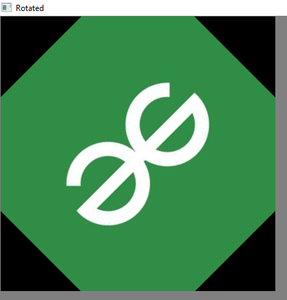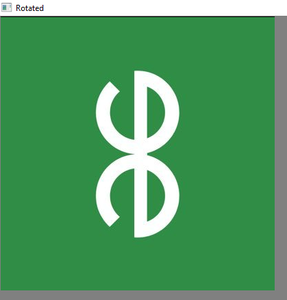Related Articles
How to rotate an image using Python?
• Last Updated : 23 Dec, 2020

In this article, let’s see how to rotate an Image using Python. By Image Rotation, the image is rotated about its center by a specified number of degrees. The rotation of an image is a geometric transformation. It can be done either by Forward Transformation (or) Inverse Transformation.

Here Image Processing Library with Pillow uses Inverse Transformation. If the Number Of Degrees Specified for Image Rotation is Not an Integer Multiple of 90 Degrees, then some Pixel Values Beyond Image Boundaries i.e Pixel values lying outside the Dimension of the image. Such Values will not be displayed in the output image.

Method:1 Using Image Processing Library Pillow

## Python3

 `# import the Python Image ``# processing Library``from` `PIL ``import` `Image`` ` `# Giving The Orginal image Directory ``# Specified``Original_Image ``=` `Image.``open``(``"./gfgrotate.jpg"``)`` ` `# Rotate Image By 180 Degree``rotated_image1 ``=` `Original_Image.rotate(``180``)`` ` `# This is Alternative Syntax To Rotate ``# The Image``rotated_image2 ``=` `Original_Image.transpose(Image.ROTATE_90)`` ` `# This Will Rotate Image By 60 Degree``rotated_image3 ``=` `Original_Image.rotate(``60``)`` ` `rotated_image1.show()``rotated_image2.show()``rotated_image3.show()`

Output:This is Image is Rotated By 180 DegreeThis Image Is Rotated By 60 DegreeThis Image Is Rotated By 90 Degree

The rotate() method of Python Image Processing Library Pillow Takes the number of degrees as a parameter and rotates the image in Counter Clockwise Direction to the number of degrees specified.

Method 2: Using Open-CV to rotate an image by an angle in Python

This is common that everyone knows that Python Open-CV is a module that will handle real-time applications related to computer vision. Open-CV works with image processing library imutils which deals with images. The imutils.rotate() function is used to rotate an image by an angle in Python.

## Python3

 `import` `cv2  ``# importing cv``import` `imutils`` ` ` ` `# read an image as input using OpenCV``image ``=` `cv2.imread(r``".\gfgrotate.jpg"``)`` ` `Rotated_image ``=` `imutils.rotate(image, angle``=``45``)``Rotated1_image ``=` `imutils.rotate(image, angle``=``90``)`` ` `# display the image using OpenCV of``# angle 45``cv2.imshow(``"Rotated"``, Rotated_image)`` ` `# display the image using OpenCV of ``# angle 90``cv2.imshow(``"Rotated"``, Rotated1_image)`` ` `# This is used for To Keep On Displaying``# The Image Untill Any Key is Pressed``cv2.waitKey(``0``)`

Output:Image Rotated Using Open-CV in 45 DegreeImage Rotated Using Open-CV in 90 Degree

Even this Open-CV Rotates the image in Counter Clockwise direction to the number of degrees specified

Attention geek! Strengthen your foundations with the Python Programming Foundation Course and learn the basics.

To begin with, your interview preparations Enhance your Data Structures concepts with the Python DS Course.

My Personal Notes arrow_drop_up